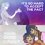# Ernest Rutherford's Gold Foil Experiment

On conducting this famous experiment Rutherford observed: 1. Some of the alpha rays went through the gold foil normally. 2. Some of them were deflected by severe angles i.e. greater than $90^\circ$. 3. A few that is $\frac{1}{20000}$ bounced back with an angle of $180^\circ$. I can understand that the first two observations were made with the help of a zinc sulphide screen but what about the third observation. How did he observe the bouncing back of alpha rays. Please answer this query. Thanks in advance.Note by Soham Dibyachintan
7 years, 3 months ago

This discussion board is a place to discuss our Daily Challenges and the math and science related to those challenges. Explanations are more than just a solution — they should explain the steps and thinking strategies that you used to obtain the solution. Comments should further the discussion of math and science.

When posting on Brilliant:

• Use the emojis to react to an explanation, whether you're congratulating a job well done , or just really confused .
• Ask specific questions about the challenge or the steps in somebody's explanation. Well-posed questions can add a lot to the discussion, but posting "I don't understand!" doesn't help anyone.
• Try to contribute something new to the discussion, whether it is an extension, generalization or other idea related to the challenge.

MarkdownAppears as
*italics* or _italics_ italics
**bold** or __bold__ bold
- bulleted- list
• bulleted
• list
1. numbered2. list
1. numbered
2. list
Note: you must add a full line of space before and after lists for them to show up correctly
paragraph 1paragraph 2

paragraph 1

paragraph 2

[example link](https://brilliant.org)example link
> This is a quote
This is a quote
    # I indented these lines
# 4 spaces, and now they show
# up as a code block.

print "hello world"
# I indented these lines
# 4 spaces, and now they show
# up as a code block.

print "hello world"
MathAppears as
Remember to wrap math in $$ ... $$ or $ ... $ to ensure proper formatting.
2 \times 3 $2 \times 3$
2^{34} $2^{34}$
a_{i-1} $a_{i-1}$
\frac{2}{3} $\frac{2}{3}$
\sqrt{2} $\sqrt{2}$
\sum_{i=1}^3 $\sum_{i=1}^3$
\sin \theta $\sin \theta$
\boxed{123} $\boxed{123}$

Sort by:

It must have been a bit tricky for Rutherford, but he simply placed the detector directly in front of the gold foil, i.e., about where the source of the alpha rays was. Maybe he was not able to measure at exactly 180 degrees back, but close enough to get the idea.

Had he been using light instead of alpha rays, this can be done with a partially silvered mirror.

- 7 years, 3 months ago

Thanks a lot sir.

- 7 years, 3 months ago

- 7 years, 3 months ago

Since the size of the nucleus was very small as compared to that of atom, so only very few particles striked the foil directly straight (which resulted in 180 degree bounce back).........was this your needed answer?

- 7 years, 3 months ago

The zinc sulphide screen was present all around, so even if he could not determine the exact angle, he could speculate that one of 20000 bounced back seeing the glow exactly behind the gold foil

- 7 years, 3 months ago

I'm pretty sure if, upon inspection of the zinc sulfide scintillator, you see that at angles very near $180^o$ there are a number incident alpha particles, you could assume that some of the alpha particles could have definitely been deflected at $180^o$. I see it as being comparable to showing that the limit of a multivariable function at some point in its allowable domain exists using the epsilon-delta definition of a limit.

- 7 years, 3 months ago